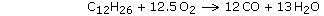## Combustion & Exhaust Velocity

Rocket Propulsion:

– Thrust

– Conservation of Momentum

– Impulse & Momentum

– Combustion & Exhaust Velocity

Specific Impulse

Rocket Engines

Power Cycles

Engine Cooling

Solid Rocket Motors

Monopropellant Engines

Staging

The combustion process involves the oxidation of constituents in the fuel that are capable of being oxidized, and can therefore be represented by a chemical equation. During a combustion process the mass of each element remains the same. Consider the reaction of methane with oxygenThis equation states that one mole of methane reacts with two moles of oxygen to form one mole of carbon dioxide and two moles of water. This also means that 16 g of methane react with 64 g of oxygen to form 44 g of carbon dioxide and 36 g of water. All the initial substances that undergo the combustion process are called the reactants, and the substances that result from the combustion process are called the products.

The above combustion reaction is an example of a stoichiometric mixture, that is, there is just enough oxygen present to chemically react with all the fuel. The highest flame temperature is achieved under these conditions, however it is often desirable to operate a rocket engine at a “fuel-rich” mixture ratio. Mixture ratio is defined as the mass flow of oxidizer divided by the mass flow of fuel.

Consider the following reaction of kerosene(1) with oxygen,Given the molecular weight of C12H26 is 170 and that of O2 is 32, we have a mixture ratio ofwhich is typical of many rocket engines using kerosene, or RP-1, fuel.

The optimum mixture ratio is typically that which will deliver the highest engine performance (measured by specific impulse), however in some situations a different O/F ratio results in a better overall system. For a volume-constrained vehicle with a low-density fuel such as liquid hydrogen, significant reductions in vehicle size can be achieved by shifting to a higher O/F ratio. In that case, the losses in performance are more than compensated for by the reduced fuel tankage requirement. Also consider the example of bipropellant systems using NTO/MMH, where a mixture ratio of 1.67 results in fuel and oxidizer tanks of equal size. Equal sizing simplifies tank manufacturing, system packaging, and integration.

As we have seen previously, impulse thrust is equal to the product of the propellant mass flow rate and the exhaust gas ejection speed. The ideal exhaust velocity is given bywhere k is the specific heat ratio, R’ is the universal gas constant (8,314.51 N-m/kmol-K in SI units, or 49,720 ft-lb/(slug-mol)-oR in U.S. units), Tc is the combustion temperature, M is the average molecular weight of the exhaust gases, Pc is the combustion chamber pressure, and Pe is the pressure at the nozzle exit.

Specific heat ratio(2) varies depending on the composition and temperature of the exhaust gases, but it is usually about 1.2. The thermodynamics involved in calculating combustion temperatures are quite complicated, however, flame temperatures generally range from about 2,500 to 3,600 oC (4,500-6,500 oF). Chamber pressures can range from about about 7 to 250 atmospheres. Pe should be equal to the ambient pressure at which the engine will operate, more on this later.

From equation (1.22) we see that high chamber temperature and pressure, and low exhaust gas molecular weight results in high ejection velocity, thus high thrust. Based on this criterion, we can see why liquid hydrogen is very desirable as a rocket fuel.

```PROBLEM 1.5

A rocket engine burning liquid oxygen and kerosene operates at a mixture ratio of
2.26 and a combustion chamber pressure of 50 atmospheres.  If the nozzle is expanded
to operate at sea level, calculate the exhaust gas velocity relative to the rocket.

SOLUTION,

Given:  O/F = 2.26
Pc = 50 atm
Pe = Pa = 1 atm

From LOX/Kerosene Charts we estimate,

Tc = 3,470 K
M = 21.40
k = 1.221

Equation (1.22),

Ve = SQRT[ (2 × k / (k - 1)) × (R' × Tc / M) × (1 - (Pe / Pc)(k-1)/k) ]
Ve = SQRT[ (2 × 1.221 / (1.221 - 1)) × (8,314.51 × 3,470 / 21.40) × (1 - (1 / 50)(1.221-1)/1.221) ]
Ve = 2,749 m/s```

It should be pointed out that in the combustion process there will be a dissociation of molecules among the products. That is, the high heat of combustion causes the separation of molecules into simpler constituents that are then capable of recombining. Consider the reaction of kerosene with oxygen. The true products of combustion will be an equilibrium mixture of atoms and molecules consisting of C, CO, CO2, H, H2, H2O, HO, O, and O2. Dissociation has a significant effect on flame temperature.

If you wish to learn more about the thermodynamics of rockets engines, please consider reading the appendix Rocket Thermodynamics.

Or you can skip all the science and just look up the numbers you need. See Propellant Combustion Charts to find optimum mixture ratio, adiabatic flame temperature, gas molecular weight, and specific heat ratio for some common rocket propellants.

 (1) In dealing with combustion of liquid hydrocarbon fuels it is convenient to express the composition in terms of a single hydrocarbon, even though it is a mixture of many hydrocarbons. Thus gasoline is usually considered to be octane, C8H18, and kerosene is considered to be dodecane, C12H26.(2) Specific heat, or heat capacity, represents the amount of heat necessary to raise the temperature of one gram of a substance one degree C. Specific heat is measured at constant-pressure, CP, or at constant-volume, CV. The ratio CP/CV is called the specific heat ratio, represented by k or.

This entry was posted in Rocket Propulsion and tagged , , . Bookmark the permalink.

### One Response to Combustion & Exhaust Velocity

1. TWA517 says:

I’ve tried problem 1.5 and example problem 1.22 with the numbers given and it doesn’t work. The first 2 terms work out, but the 1-(Pe/Pc) with exponent breaks the problem….any thoughts
P.S. even my 7th and 8th graders could not get it to work.